# Schrödinger Wave Equation: Derivation & Explanation

Contents

## What is the Schrodinger Equation

The Schrödinger equation (also known as Schrödinger’s wave equation) is a partial differential equation that describes the dynamics of quantum mechanical systems via the wave function. The trajectory, the positioning, and the energy of these systems can be retrieved by solving the Schrödinger equation.

All of the information for a subatomic particle is encoded within a wave function. The wave function will satisfy and can be solved by using the Schrodinger equation. The Schrodinger equation is one of the fundamental axioms that are introduced in undergraduate physics. It is also increasingly common to find the Schrödinger equation being introduced within the electrical engineering syllabus in universities as it is applicable to semiconductors.

Unfortunately, it is only stated as a postulate in both cases and never derived in any meaningful way. This is quite dissatisfying as nearly everything else taught in undergraduate quantum physics is built upon this foundation. In this article, we will derive the equation from scratch and I’ll do my best to show every step taken.

Interestingly enough, the arguments we will make are the same as those taken by Schrödinger himself so you can see the lines of thinking a giant was making in his time. As a reminder, here is the time-dependent Schrödinger equation in 3-dimensions (for a non-relativistic particle) in all of its beauty:

## Quantum Physics and Waves

Everyone likes to bag out classical physics – but it served us pretty well for quite a while (think Newtonian mechanics, Maxwell’s equations, and special relativity).

However, as shown in our previous articles, experimental results in the turn of the century weren’t looking too flash when compared to the known physics at the time. Our articles on the double slit experiment and to some degree the photoelectric effect are experimental results that didn’t match up well with the known understanding of the time.

But why? To put it simply, in classical physics there exist two entities, particles and waves. The features of both of these entities can be described as follows:

• Particles: localized bundles of energy and momentum with mass.
• Waves: disturbances spread over space-traveling over time. They can be described with a wave function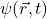that describes the wave over space and time.

This brings us to the surprising results found in our Photoelectric Emission article. We found that the electron shows both of these properties. This was in complete contradiction with the known understanding of the time as the two entities were considered mutually exclusive.

Insane right? About this time, some really influential figures in physics started realizing that there was a gap in knowledge, and a large breakthrough came about when Louis de Broglie associated a momentum (for a particle) to a wavelength (for waves) given by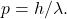Also, from Photoelectric Emission we know that there energy absorption and emission of photons (still unsure whether particle or wave) have energy given by:Whereand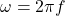. We are now at the exact same stage Schrödinger was before deriving his famous equation. But where do we begin? Well, we know that the electrons and photons are showing wave-like and particle-like behavior. There wouldn’t be anything wrong with starting with a universal equation that all waves should obey and then introducing particle physics on top to see if there is a result.

## How to derive the Wave Equation

The disturbanceobeys the wave equation. Remember, the electron displays wave-like behavior and has an electromagnetic charge. Therefore, for now, let us just look at electromagnetic fields. In this scenario, Maxwell’s equations apply and here they are in all of their glory: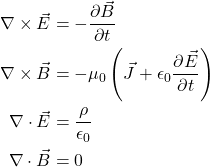Where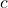is the speed of light in a vacuum,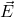is the electric field and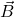is the magnetic field. The first equation above is the basis of electric generators, inductors, and transformers and is the embodiment of Faraday’s Law.

Also, one of the implications from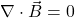is that no magnetic monopoles exist. Understanding the derivation of these equations and the physical meaning behind them makes for a well-rounded engineer. Now, let us derive the equation that any electromagnetic wave must obey by applying a curl to Equation 4: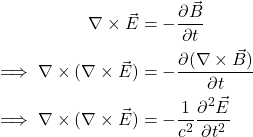Now we can leverage a very familiary (and easily proven) vector identity: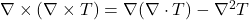whereis some placeholder vector. Applying to our little equation now:The result we have here is the electromagnetic wave equation in 3-dimensions. This equation is manifested not only in an electromagnetic wave – but has also shown in up acoustics, seismic waves, sound waves, water waves, and fluid dynamics.

## How to Derive the Schrödinger Equation

### Plane Wave Solutions to the Wave Equation

Beginning with the wave equation for 1-dimension (it’s really easy to generalize to 3 dimensions afterward as the logic will apply in alland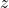dimensions.):This is, in reality, a second-order partial differential equation and is satisfied with plane wave solutions: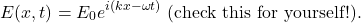Where we know from normal wave mechanics that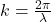and. Now, let us make use of the work from Einstein and Compton and substitute in the fact that the energy of a photon is given by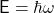and from de-Broglie that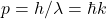. We can further massage our plane wave solution to:This is the plane wave equation describing a photon. Let’s substitute this equation into our wave equation and see what we find!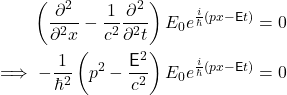In other words,which is great because we know from special relativity that the total energy for a relativistic particle with mass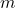is: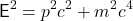And we’ve only been dealing with the photon so far which has no mass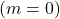! So let’s expand our understanding and apply the total relativistic energy for a particle with mass (like the electron for example) and change the name of our equation tobecause we’re ballers.Now this equation came straight from substituting the plane wave equation for a photon into the wave equation. However, since we now want the energy to solve the total relativistic energy for a particle with mass, we need to change the wave equation slightly. This is because the wave equation shouldn’t fully apply to our newwhich describes particles and waves. We can now backsolve for an operator to get the equation above, and it’s given by: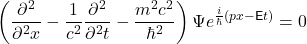### Solving for Particles with Mass in the Wave Equation

We now want to make a few approximations on the full energy we just described by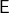for a particle with momentum and mass. Let’s just rearrange the formula slightly so we can use some approximations.The whole point of this manipulation is to get the equation in the form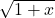because if we take a Taylor Series expansion of this equation we get:When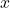is small, the only part that remains in the Taylor expansion is theterm. In our energy formula,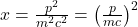. We can take advantage of the fact thatfor anything that isn’t traveling at the speed of light (please find me if you do find anything that doesn’t satisfy this)! So this term actually reduces to:WhereIs the normal kinetic energy we see from high school physics. Now back to the wave function from before, let’s now input in this new information and see what we end up with: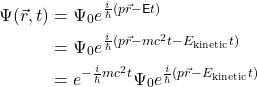The reason we have now split the two terms it that the first term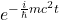(just based on the speed of light again) will be significantly more oscillatory to that of the second term and doesn’t necessarily describe the particle-wave entity we are after. So to solidify this difference, let’s now establish that: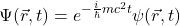Where we have now defined: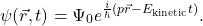Let’s now take the first and second partial derivatives of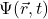and see what we end up with. The first: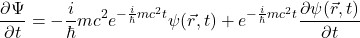and the second:We should keep in mind that the last term with the second partial derivative is quite small because of the fact that there is noterm carrying the order of magnitude, and therefore by approximation, the actual second derivative is given by: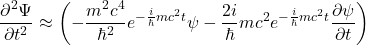The sneaky reason we took these two partial derivatives was so that we could impute them into this equation describing the wave function earlier: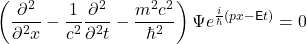But before we can do that, let’s rearrange this formula and we’ll end up with an equation called the Klein-Gordon equation: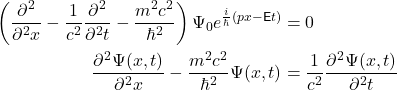Now we can easily generalize this to 3-dimensions by turning this equation into a vector equation (all the steps we took to derive this formula will apply for alland.)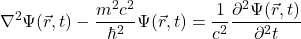This equation is known as the Klein-Gordon equation for a free particle. This equation is relativistic as it’s energy term doesn’t make assumptions we did with the little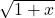Taylor expansion.

Now, let’s simplify the Klein-Gordon equation (going back down to 1-D and applying our new energy formula) and we’ll arrive at the long-awaited Schrödinger Equation: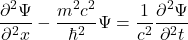Let’s put in our new wave function given by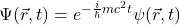where we know what the first and second derivatives with respect to time look like: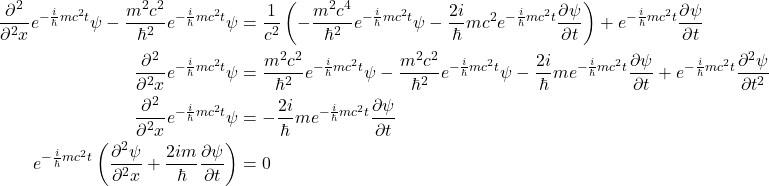Now all we need to do is a simple rearrange to obtain the Schrödinger Equation in three dimensions (note that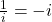):Where the argument can be made by noting the similarity of the classical Hamiltonian that the term on the right-hand side of the equation describes the total energy of the wave function.

In our derivation, we assumed that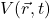is 0 and that only the kinetic energy was taken into account. We know that the potential is purely additive with respect to its spatial variations and therefore, the full Schrödinger Equation in three dimensions with potential is given by: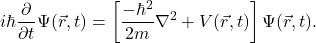That’s it! There we have it, this article has derived the full Schrodinger equation for a non-relativistic particle in three dimensions. If you’ve liked this post and would like to see more like this, please email us to let us know.

## Citations

Want To Learn Faster? 🎓
Get electrical articles delivered to your inbox every week.
No credit card required—it’s 100% free.1.